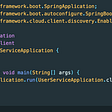# All Elements in Two Binary Search Trees — Day 108(Python)

Today’s question is from the daily leetcode challenge. Let us look into the problem statement.

## 1305. All Elements in Two Binary Search Trees

Given two binary search trees `root1` and `root2`, return a list containing all the integers from both trees sorted in ascending order.

Example 1:

`Input: root1 = [2,1,4], root2 = [1,0,3]Output: [0,1,1,2,3,4]`

Example 2:

`Input: root1 = [1,null,8], root2 = [8,1]Output: [1,1,8,8]`

Constraints:

• The number of nodes in each tree is in the range `[0, 5000]`.
• `-105 <= Node.val <= 105`

One way to solve this problem would be, to add all the values of the nodes from both the trees into a list, and then can use python’s inbuilt sort function to sort the list.

`class Solution:    def getAllElements(self, root1: TreeNode, root2: TreeNode) -> List[int]:        def traverse(root, output):            if root == None:                return output            traverse(root.left, output)            output.append(root.val)            traverse(root.right, output)            return output        output = traverse(root1, [])        output = traverse(root2, output)        return sorted(output)`

Complexity Analysis

Time Complexity

We are creating a list of node values from both the trees and then using python’s inbuilt sort function to sort our list. Hence the time complexity is (M+N) log(M+N), where M and N are the number of nodes in both the trees.

Space Complexity

We are creating a list of node values from both the trees, hence the space complexity is O(M+N).

Can we not use the sort function? Since we are given binary search trees, we can use in-order traversal and get a sorted list. If you need to read more about the Binary Search tree, you can follow this link.

Once we have both the sorted list, we can compare the first element in both the lists and the add the smaller among two elements to our output list. We will keep doing this until both our sorted lists are empty.

`class Solution:    def getAllElements(self, root1: TreeNode, root2: TreeNode) -> List[int]:        output = []        def inorder(root, my_list):            if root == None:                return my_list            inorder(root.left, my_list)            my_list.append(root.val)            inorder(root.right, my_list)            return my_list        root1_l = inorder(root1, [])        root2_l = inorder(root2, [])        while(root1_l and root2_l):            if root1_l <= root2_l:                output.append(root1_l.pop(0))            else:                output.append(root2_l.pop(0))        while(root1_l):            output.append(root1_l.pop(0))        while(root2_l):            output.append(root2_l.pop(0))        return output`

Complexity Analysis

Time Complexity

We are creating two sorted list by traversing through both the binary search trees. The traversal takes O(M+N), where M and N are the number of nodes in both the trees. After getting the sorted list, we are comparing the elements in both the list, which takes O(M+N). Hence time complexity is O(M+N).

Space Complexity

We are creating a list of node values from both the trees, hence the space complexity is O(M+N).

--

--

--

## More from Annamariya Tharayil

Software Engineer. Find me @ www.linkedin.com/in/annamariya-jt

Love podcasts or audiobooks? Learn on the go with our new app.

## Recommended from Medium## Towards Container-Based Development 🐬🐬🐬## Autonomous Wireless Network — Operational Aspects## How to build a visual recognition mobile app in 2 days with React-Native and Clarifai## User Service Skeleton## Release 0.4, My Experiences## Annamariya Tharayil

Software Engineer. Find me @ www.linkedin.com/in/annamariya-jt

## Scheduling Algorithms In Operating System Explained!## Python-P02-Prepare the Work(Py)station## Koko Eating Bananas — Day 106(Python)